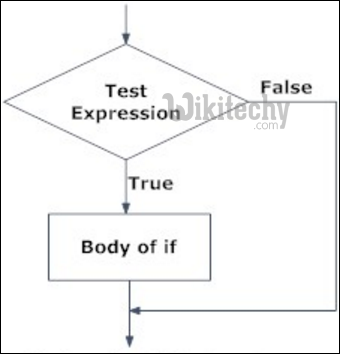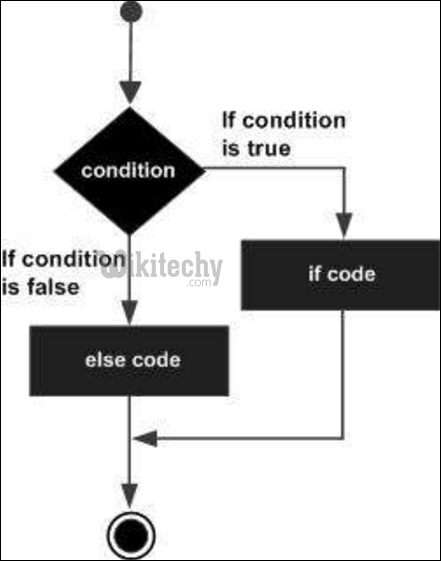# python tutorial - Python If Else | Python If Else Statements - learn python - python programming## Python if Statement

• An if statement is a programming conditional statement that, if proved true, performs a function or displays information.
• Basic example of an if statement, not specific to any particular programming language.Learn Python - Python tutorial - python ifelse - Python examples - Python programs

## Python if Statement Flowchart## Syntax

• In the example above, if the value of X were equal to any number less than 10, the program would print, or display, "Hi Wikitechy" on the console when the script is run.

## Python If Else Statements

• An else statement can be combined with an if statement.
• An else statement contains the block of code that executes if the conditional expression in the if statement resolves to 0 or a FALSE value.
• The else statement is an optional statement and there could be at most only one else statement following if.

## Syntax## Output:

Wikitechy tutorial site provides you all the learn python , python wiki , python loop , python with , python switch , print python , python import , python os , learning python book , python book online , python programming training course , python programming course online , online python training course , python training online